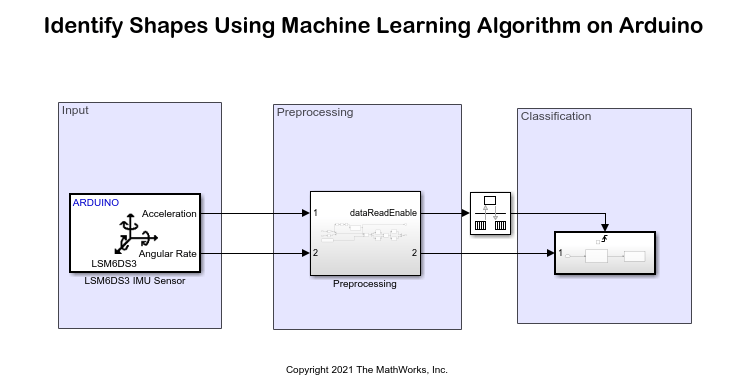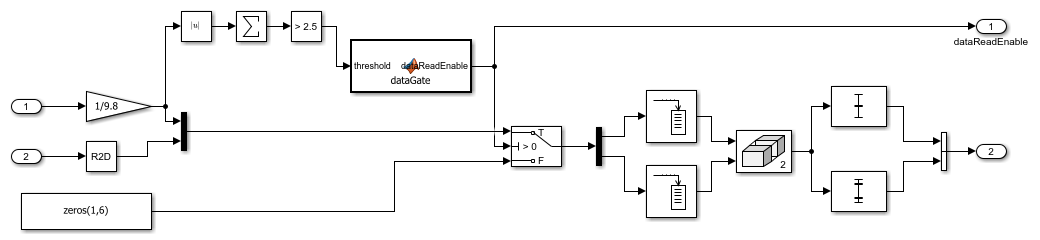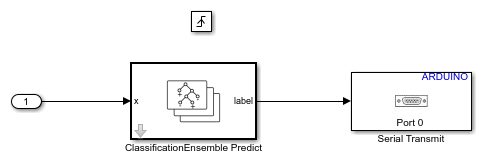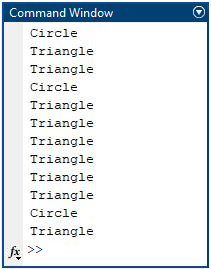Identify Shapes Using Machine Learning Algorithm on Arduino

This example shows how to use the Simulink® Support Package for Arduino® Hardware to identify shapes such as triangles and circles using a machine learning algorithm. The example is deployed on an Arduino Nano 33 IoT hardware board that uses an onboard LSM6DS3 IMU Sensor to identify shapes. The output from the machine learning algorithm, after identifying shapes, is transmitted to the serial port to publish the output in the MATLAB® Command Window.

Required Hardware

• Arduino Nano 33 IoT board

• USB cable

Hardware Setup

Connect the Arduino Nano 33 IoT board to the host computer using the USB cable.

Prepare Data Set for Training Machine Learning Algorithm

In this example, you can use the pretrained data to identify a circle or a triangle in the shapes_training_data.mat file. Alternatively, you can train data using the preconfigured MATLAB code in the capture_training_data.m file and store the trained data in the shapes_training_data.mat file.

Run this command in the MATLAB Command Window to load the shapes_training_data.mat file.

This MAT file contains 119 data samples that are read from an accelerometer and a gyroscope. These 119 samples are grouped into 100 frames, where each frame represents a hand gesture. Each frame has six values that are obtained from the X, Y, and Z axes of the accelerometer and gyroscope. A total of 11,900 observations are stored in the data set for two different shapes, circle and triangle.

Follow this procedure to train and store the trained data in the MAT file shapes_training_data.mat.

1. In the MATLAB Command Window, run this command to edit the gr_script_shapes.m file.

edit gr_script_shapes;

2. Configure these parameters.

a. Specify the acceleration threshold in the accelerationThreshold parameter. In this example, the threshold is set to 2.5.

b. Create an lsm6ds3 (MATLAB Support Package for Arduino Hardware) object and specify the number of samples read in a single execution of the read function. In this example, the parameter is set to 119.

c. Specify the number of frames to be captured per gesture in the while loop. In this example, 100 frames are captured per gesture.

3. To capture hand movement while drawing a circle, run this command in the MATLAB Command Window.

circle_data = shapes_training_data;

4. Hold the Arduino hardware in the palm of your hand and draw the shape of a circle in the air. To create a data set of 100 frames for a circle, draw a circle 100 times. Note that the value of the Gesture no. increments in the MATLAB Command Window every time you draw a circle.

5. To capture the hand movement while drawing a triangle, run this command in the MATLAB Command Window.

triangle_data = shapes_training_data;

6. Hold the Arduino hardware in the palm of your hand and draw the shape of a triangle in the air. To create a data set of 100 frames for a triangle, draw a triangle 100 times. Note that the value of the Gesture no. increments in the MATLAB Command Window every time you draw a triangle.

7. When you create your own data set for a circle and a triangle, use the same name for the MAT file in the gr_script_shapes MATLAB code file. The gr_script_shapes MATLAB code file is used to preprocess and train the data set for a circle and a triangle, train the machine learning algorithm with the data set, and evaluate its performance to accurately predict these shapes.

Use the MATLAB code in the gr_script_shapes file and then deploy the Simulink model on the Arduino hardware.

Extract Feature

Extract the features by taking the mean and the standard deviation of each column in a frame. Doing so results in a 100-by-12 matrix of observations for each gesture.

Nframe = 100;
for ind = 1:Nframe
featureC1(ind,:) = mean(circledata{ind});
featureC2(ind,:) = std(circledata{ind});
featureT1(ind,:) = mean(triangledata{ind});
featureT2(ind,:) = std(triangledata{ind});
end
X = [featureC1,featureC2;
featureT1,featureT2;
zeros(size(featureT1)),zeros(size(featureT2))];

Prepare Data

This example uses 80% of the observations to train a model that classifies two types of shapes and 20% of the observations to validate the trained model. Use cvpartition (Statistics and Machine Learning Toolbox) to hold out 10% of the data for the test data set.

rng('default') % For reproducibility
Partition = cvpartition(Y,'Holdout',0.20);
trainingInds = training(Partition); % Indices for the training set
XTrain = X(trainingInds,:);
YTrain = Y(trainingInds);
testInds = test(Partition); % Indices for the test set
XTest = X(testInds,:);
YTest = Y(testInds);

Train Decision Ensemble at Command Line

Train a classification model.

template = templateTree(...
'MaxNumSplits', 399);
ensMdl = fitcensemble(...
XTrain, ...
YTrain, ...
'Method', 'Bag', ...
'NumLearningCycles', 20, ...
'Learners', template, ...
'ClassNames', [1; 2; 3]);

Perform a five-fold cross-validation for classificationEnsemble and compute the validation accuracy.

partitionedModel = crossval(ensMdl,'KFold',5);
validationAccuracy = 1-kfoldLoss(partitionedModel);
ans =
validationAccuracy = 0.9833

Evaluate Performance on Test Data

Evaluate the performance of the classification model on the test data set.

testAccuracy = 1-loss(ensMdl,XTest,YTest)
ans =
testAccuracy = 1

The trained model accurately classifies 100% of the shapes in the test data set. This result confirms that the trained model does not overfit the training data set.

Prepare Simulink Model and Calibrate Parameters

Use gr_script_shapes.m file as a Simulink model initialization function.

To open the Simulink model, run this command in the MATLAB Command Window.

open_system('arduino_machinelearning_shapes')The Arduino Nano 33 IoT board has an onboard LSM6DS3 IMU sensor that measures linear acceleration and angular velocity along the X, Y, and Z axes. Configure these parameters in the Block Parameters dialog box of the LSM6DS3 IMU Sensor block.

1. Set the I2C address of the sensor to 0x6A to communicate with the accelerometer and gyroscope peripherals of the sensor.

2. Select the Acceleration (m/s^2) and Angular velocity (rad/s) output ports.

3. Set the Sample time to 0.01.

The 1-by-3 acceleration and angular velocity vector data is collected from the LSM6DS3 IMU sensor at the sample time you specify in the Block Parameters dialog box. The Preprocessing subsystem then preprocesses this data.

To open the subsystem, run this command in the MATLAB Command Window.

open_system('arduino_machinelearning_shapes/Preprocessing')The acceleration data is first converted from m/s^2 to g. The absolute values are then summed and for every 119 data values that are greater than a threshold of 2.5 g, the dataReadEnable parameter in the MATLAB Function block becomes logically true. A logical true value acts as a trigger to the Triggered subsystem in the Classification area.

The angular velocity data is converted from radians to degrees. The acceleration and angular velocity data is multiplexed and given as an input to the switch. For a data value greater than 0, the buffer stores the valid 119 gesture values corresponding to a circle and a triangle. For data values less than zero, which indicates that no hand gesture is detected, a series of [1,6] zeros are sent to the output to match the combined acceleration and angular velocity data.

Configure this parameter in the Block Parameters dialog box of the Buffer block.

1. Set the Output buffer size parameter to 119.

Features are extracted by calculating the mean and the standard deviation values of each column in a frame that results in a 100-by-12 matrix of observations for each gesture. These extracted features are further passed as an input to the Triggered subsystem in the Classification area.

To open the subsystem, run this command in the MATLAB Command Window.

open_system('arduino_machinelearning_shapes/Triggered Subsystem')The Rate Transition block transfers data from the output of the Preprocesssing subsystem operating at one rate to the input of the Triggered subsystem operating at a different rate.

The ClassificationEnsemble Predict (Statistics and Machine Learning Toolbox) block is a library block from Statistics and Machine Learning Toolbox™ that classifies gestures using the extracted features. The block uses the ensMdl machine learning model to identify shapes. This block outputs the predicted class label. The output is either a circle or a triangle.

The Serial Transmit block parameters are configured to their default values.

1. On the Hardware tab of the Simulink model, in the Mode section, select Run on board and then click Build, Deploy & Start.

2. For easy analysis of the hand movement data being recognized by the machine learning algorithm, run the following script in the MATLAB Command Window and read data on the Arduino serial port.

device = serialport(serial_port,9600);
while(true)
if rxData == 1
disp('Circle');
elseif rxData == 2
disp('Triangle');
end
end

You can also run this command in the MATLAB Command Window and edit the read_shapes_data_from_device.m file.

3. Replace the port parameter with the actual com port of your Arduino board. The gesture detected by the machine learning algorithm is displayed on the Arduino serial port at a baud rate of 9600.

4. Hold the hardware in the palm of your hand and draw shapes in the air. Observe the output in the MATLAB Command Window.Other Things to Try

• Train the machine learning algorithm to identify shapes such as squares, pentagons, and numbers from 0 to 9.

• Use a machine learning algorithm other than classificationEnsemble and identify various shapes and numbers.